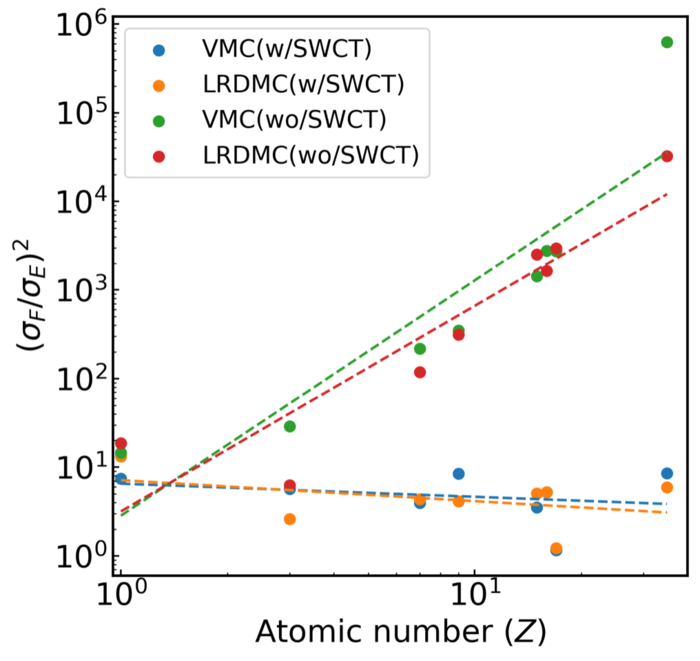# Increasing the Accuracy of Atomic Force Calculations with the Space-Warp Coordinate TransformationThis figure shows the ratios between the force and energy variances evaluated for several molecules. We found that the ratio scales as Z2.5 without the SWCT in the QMC calculations, while the ratio is independent of Z with the SWCT both in the VMC and LRDMC calculations, indicating that the computational cost of QMC forces with respect to Z is no worse than that of energy. Indeed, the accessible system size is not affected by QMC force calculations when the SWCT variance-reduction technique is applied.

Atomic forces are primarily responsible for the motion of atoms and their versatile arrangement patterns, which is unique for different types of materials. Atomic simulation methods are a popular choice for the calculation of these forces, the understanding of which can vastly enhance existing knowledge on how to improve a material’s function at an atomic level.

Quantum Monte Carlo (QMC) methods are high-precision, state-of-the-art simulation methods, which are used to obtain many-body wave functions, essential for the calculation of atomic forces. These methods have recently gained importance, owing to their ability to simulate the microscopic behavior of matter with extremely high accuracy, and for overcoming the disadvantages of conventional simulation methods.

The two major QMC methods are the variational Monte Carlo (VMC) method, and the fixed node diffusion Monte Carlo (FNDMC) method. FNDMC methods provide more accurate results than the VMC methods, since the latter is highly dependent on the quality of the trial wave function to calculate necessary parameters, such as the ground state wave function of atoms.

While QMC methods are reliable for the calculation of ground-state energies of atoms, they result in very high calculation costs for atomic forces of elements with higher atomic numbers. Moreover, there is no consensus among the research community on an efficient algorithm for QMC force evaluation, which should ideally scale well with number of electrons and atomic number. This leads to a few inaccuracies in atomic force calculations for elements with high atomic numbers.

To address this shortcoming, a group of researchers led by Assistant Professor Kousuke Nakano from the Japan Advanced Institute of Science and Technology, recently proposed a space-warp coordinate transformation (SWCT) method, to reduce the calculation costs incurred while calculating atomic forces.

For the purpose of this study, which was published in The Journal of Chemical Physics, the team utilized a “TurboRVB” software developed by Dr. Nakano et al., which implemented a ‘lattice regularized’ version of the FNDMC method, also known as the LRDMC method. Using this software, they calculated the all-electron VMC and LRDMC forces, with and without the space-warp coordinate transformation, for mono and heteronuclear atom dimers, using the following atoms: H2, Li2, N2, F2, P2, S2, Cl2, and Br2. Furthermore, they calculated the LRDMC forces using Reynolds (RE) and Variational-Drift (VD) approximation methods.

An analysis of these calculations revealed that the energy surface values derived from LRDMC method gave equilibrium bond lengths and harmonic frequencies values, very close to previously derived experimental values for atomic dimers, in turn improving the corresponding VMC results.

Moreover, the LRDMC forces calculations using both RE and VD approximations led to an improvement in VMC forces, although VD approximation-based calculations resulted in high computational costs.

Our findings indicate that the application of the so-called space-warp coordinate transformation (SWCT) is essential to reduce the computational cost of forces in QMC. Specifically, the ratio of computational costs between QMC energy and forces scales as Z2.5 without the SWCT, where Z is the atomic number. In contrast, the application of the SWCT makes the ratio independent of Z”, says Dr. Nakano while discussing the findings of this study.

This is one of the most important findings of the study, suggesting that the application of SWCT methods yields a constant ratio of computational cost of force to energy, regardless of the atomic number of the material.

“The accurate forces obtained by QMC could contribute to in-silico material designs, to design important materials such as medicines and molecular catalysts”, states Dr. Nakano, while highlighting the real-life applications of this study.

Thus, the SWCT method can enhance the application of the quantum Monte Carlo method to calculate atomic forces for substances that cannot be dealt with by conventional approaches – which will provide much needed relief to researchers who rely on these conventional methods. These findings highlight the accuracy and reliability of the SWCT method, and its utility for the development of important technologies in the field of material science.